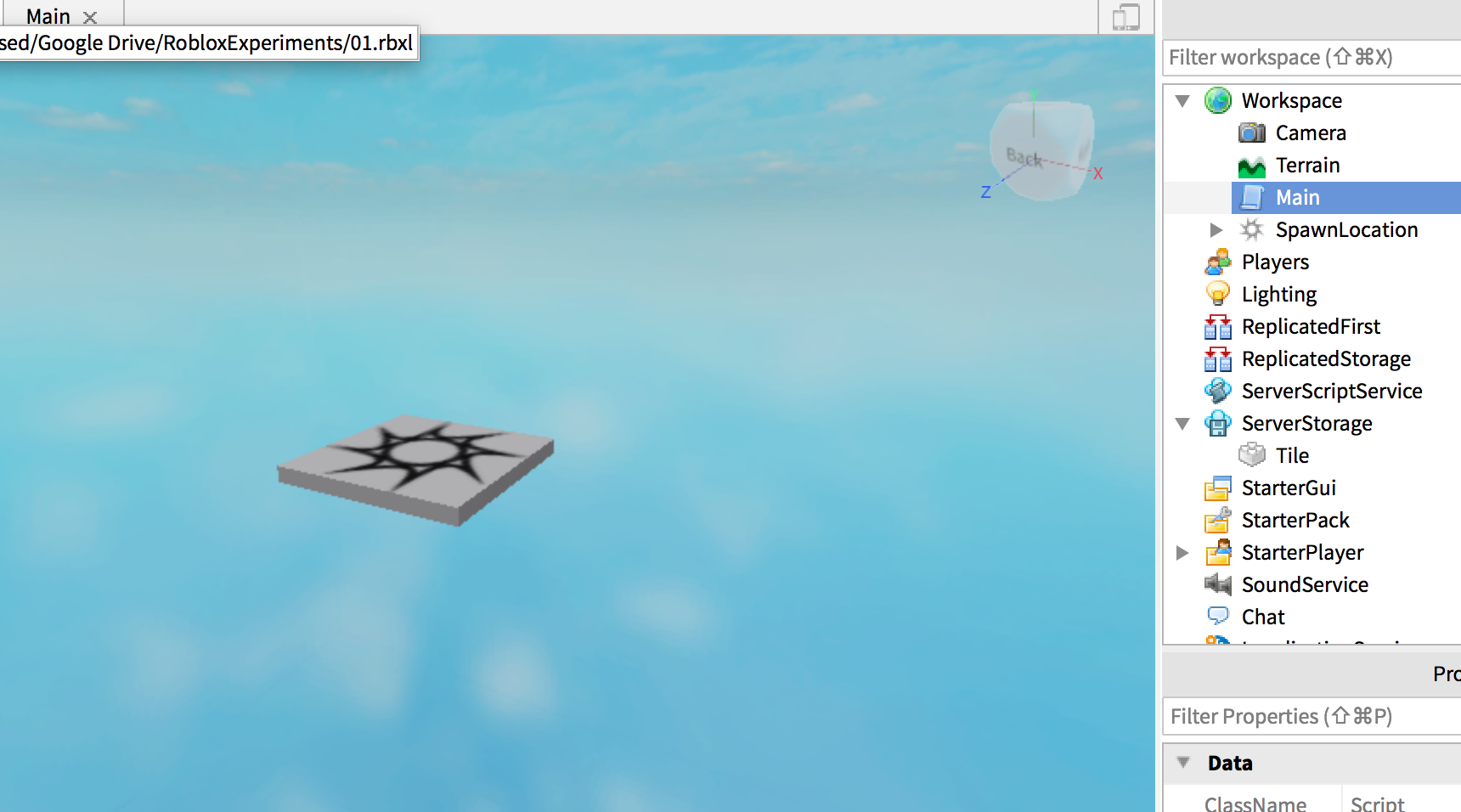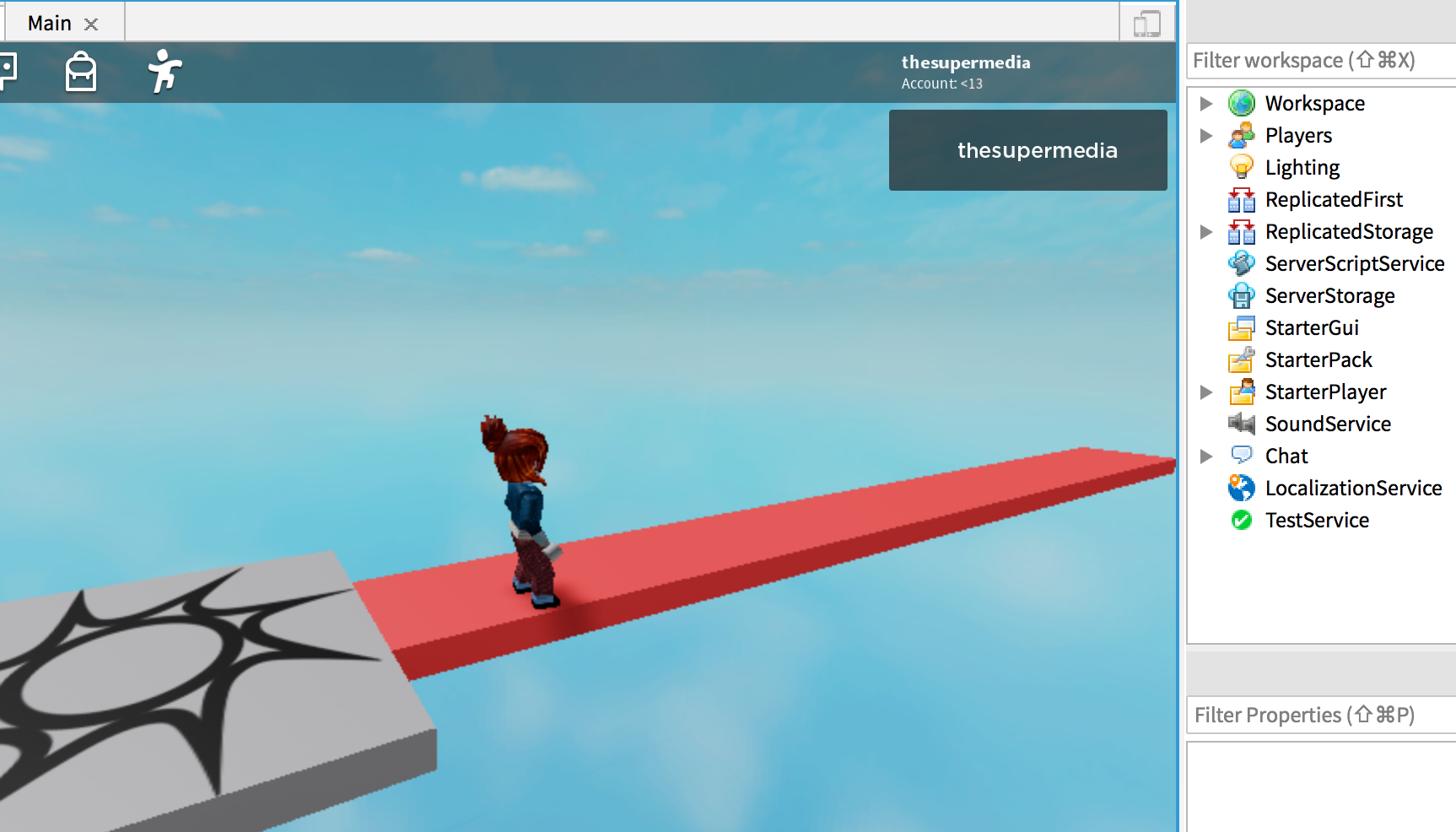We know how to create a Part with script using the `Clone` function. This code create a Part that exists in ServerStorage, a Part with name `Tile`:

``````local p = game.ServerStorage.Tile:Clone()
p.Parent = game.Workspace
p.Position = Vector3.new(0,0,-10)
``````

We can exploit the for-loop strategy to create many tiles. The following code create 6 tiles positioned along the `z` axis assuming their size is `5,1,5`

``````for count = 0, 10 do
local p = game.ServerStorage.Tile:Clone()
p.Parent = game.Workspace
p.Position = Vector3.new(0,0,-8.5 - count*5)
end
``````

The only difference is that we need to calculate the position for each, otherwise they'll be all on the same spot, that's useless.

Before running it:After hit Run: